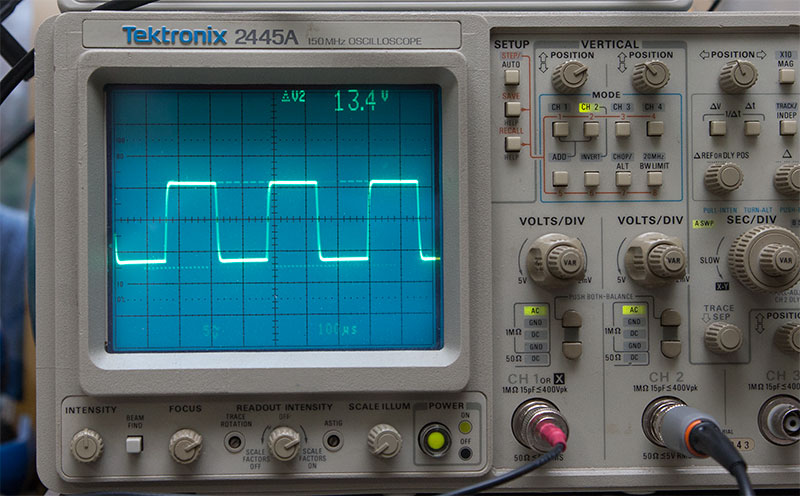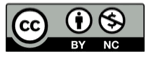# 傅立叶级数的直观解释

2018-08-19## 一般情况

$$f(t) =\displaystyle\sum_{n=0}^\infin{A_n \sin(\omega_n t)}$$

$$\omega_n= n \omega_0$$

## 一次谐波

$$\hat{f}(t) = \sin(\omega_0 t)$$

$$e^{j\omega_0 t}$$

$$\hat{f}(t) = \sin(\omega_0 t) = \mathcal{Re}(e^{j\omega_0 t})$$——苏轼《题西林壁》

## 加入三次谐波

$$f(t) =\displaystyle\sum_{n=0}^\infin{A_n \sin(n \omega_0 t)}$$

$$f(t) =\displaystyle\sum_{n=0}^\infin{A_n e^{jn\omega_0 t}}$$

$$\hat{f}(t) =A_1 e^{j\omega_0 t} + A_3 e^{j 3\omega_0 t}$$

$$\overrightarrow{a} + \overrightarrow{b} = \overrightarrow{c}$$

$$\hat{f}(t) =A_1 e^{j\omega_0 t} + A_3 e^{j 3\omega_0 t}$$

## 继续逼近(图片来源：http://www.trevormarshall.com/byte_articles/byte6.htm)

## 精彩评论